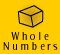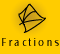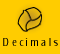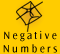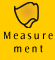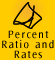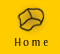| 3 types of percent computation problems |Finding the part |Finding the percent | Finding the whole | Adding percents|
| Further Applications | Calculator short cuts | Quick quiz |

We have already looked at a common application of percent in the section, Key Ideas: Percentage increases and decreases. These simple examples fall into the categories of problems below, but were introduced earlier because they are problems with which most of us are familiar.

3 types of percent computation problems

There are 3 basic types of percent computation problems which can be represented using the following expression,

r% of b is p

where r is the percent, b is the whole or base quantity and p is the part of b.

 2% of 200 is 4 r% b p percent whole part

In each type of percent problem we want to find one of the following:
- the percent (r)
- the whole (b)
- the part (p)

Using the dual-scale number line we can easily see which piece of information is missing in each type of problem. Please see Meaning and Models, Percent models and Key Ideas for more details

 For example, 12 out of 60 books on the shelf are cook books. This means 20% of the books on the shelf are cook books.finding the part (p) finding the percent (r) finding the whole (b)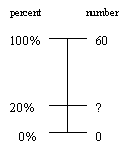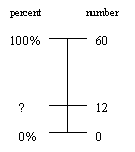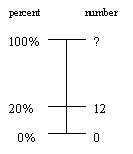What is 20% of 60 books? 12 out of 60 books is what percent? 12 is 20% of what number?

Because of the relationship of percent to common fractions and decimals there are a number of approaches we can use as a starting point to solve percent problems:

 Benchmark - Recognise common percents, such as 50% and then perform the computation if necessary. Fractions - Express the percent as a fraction, such as 54/100, and then perform the computation using fraction algorithms if necessary. Decimal - Express the percent as a decimal, such as 0.54, and then perform the computation using decimal algorithms if necessary. Unitary - Find 1% of the quantity and then perform computation if necessary. Calculator - Use short cut methods provided on some calculators

We often use the unitary or benchmark approach when working out percent problems in our heads. We will look at how we use these methods to solve each problem type below.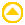Finding the part

Example 1: What is 5% of 80 kilometres?

What is r% of b? We need to find p, the part.

There are many different ways in which we could solve this problem. If we used either of the unitary or benchmark methods described below we would usually do them in our heads.

Before solving a problem it is a good idea to make an estimate of our answer so that we can check it for reasonableness when we have completed the problem. In this case we could note that 5% of 100 is 5 so we would expect our answer to be less than 5.

 Working Out Thinking 0.8 is 1% of 80 0.8 x 5 = 4 4 is 5% of 80 Unitary approach 80 is 100% of 80, so 0.8 is 1% of 80. Now we have 1% of 80 we can multiply by 5 to get 5%. 8 is 10% of 80 8 ÷ 2 = 4 4 is 5% of 80 Benchmark approach I know that 8 is 10% of 80, so to find 5% I divide 8 by 2.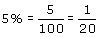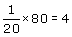4 is 5% of 80 or,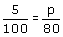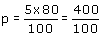p = 4 4 is 5% of 80 Fraction approach 1 We could express 5% as a fraction out of 100 and simplify it into the equivalent fraction 1/20. We know that 1/20th of 80 is 4.     Fraction approach 2 We could also say 5 out of 100 equals p out of 80 since we want to find out what part of 80 equals 5%. We then do the same to both sides of the equation to find p. 5% of 80 = 0.05 x 80 4 is 5% of 80 Decimal approach We could express 5% as a decimal, 0.05, and then multiply by 80. Using my calculator, Enter 80 Press x Enter 5 Press % Answer: 4 4 is 5% of 80 Calculator approach We could use the shortcut method provided on some calculators which have a '%' button. NOTE: the way you enter the numbers and symbols will depend on your calculator - you will need to experiment. Note also that you are not necessarily keying in a correct mathematical sentence, but rather using a shortcut method provided by your calculator.

 Example 2: Movie - simple example of finding the percentage of hard numbers using a calculator. What is 12.3% of \$1294?

We can summarise what we have been doing when using the decimal or fraction approach into a formula.

 p = (r/100) x b, where p is the part, r is the percent and b is the whole. In order to find the part we need to multiply the whole amount by the percent. Example: What is 15% of \$300? p= (15/100) x 300 p= 45 15% of \$300 is \$45

Finding the percent

Example 3: What percent is \$4 of \$80?

What percent is p of b? We need to find r, the percent.

We will first estimate our answer. 10% of \$80 is \$8 (10 x 8 = 80) so we know our answer will be less than 10%.

 Working Out Thinking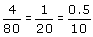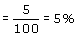4 is 5% of 80 or,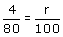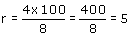4 is 5% of 80 Fraction approach 1 Our final answer will be as a fraction with a denominator of 100. How do we get there? We can simplify the fraction to a form which we can more easily convert into hundredths and then express as a percent. Fraction approach 2 We could also say 4 out of 80 equals r out of 100. We then do the same to both sides of the equation to find r.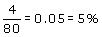4 is 5% of 80 Decimal approach We can express 4/80 as a decimal using our calculator and then express as a percent. 0.8 is 1% of 80 4 is ?% of 80 4 ÷ 0.8 = 5 4 is 5% of 80. Unitary approach 80 is 100% of 80, so 0.8 is 1% of 80. We know that 0.8 is 1% of 80 and we want to know what percent 4 is of 80. We could find out how many 0.8 's there are in 4. Four divided by 0.8 is 5. NOTE: This approach is not as suited to this problem because we still have to do a lot of working out to get our answer. We can see that all other approaches get to the answer quicker. 8 is 10% of 80 4 is 5% of 80 Benchmark approach I know that 8 is 10% of 80. 4 is half of 8, so 4 must be 5% of 80. Using my calculator, Enter 4 Press / Enter 80 Press x Enter 100 4 / 80 x 100 = 5% 4 is 5% of 80 Shortcut: Enter 4 Press / Enter 80 Press % gives 5 directly Calculator approach 4 is how many percent of 80? In the first case we are not using the '%' button but are multiplying 4/80 by 100 to give us the percent. We could use the shortcut method provided on some calculators which have a '%' button.

Example 4: Movie - finding the percent using the fraction approach.
48 people out of 360 people studied drink Brand A softdrink regularly. What percent is this?

Movie - verifying our answer to example 4 using the calculator.Finding the whole

Example 5: 4 is 5% of what number?

p is r% of what number? We need to find b, the whole.

We will first estimate our answer. 4 is 1% of 400, so 4 will be 5% of a much smaller number. We know that our answer will be a lot less than 400.

 Setting Out Thinking 4 is 10% of 40 4 is 5% of (2 x 40) 4 is 5% of 80 Benchmark approach 4 is 5% of what number. We know 4 is 10% of 40 (4 x 10 = 40), so for 4 must be 5% of two times 40.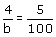4 x100 = 5 x b 5b = 400So, 4 is 5% of 80 Fraction approach We can express the problem in terms of fractions. 4 divided by some number, b, is equivalent to 5/100 (5%). We can then do the same to both sides of the equation to find b. 5% = 0.05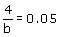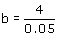b=80 4 is 5% of 80 Decimal approach We can express 5% as a decimal fraction, 0.05. 4 divided by b is equivalent to 0.05 (5%). We can then do the same to both sides of the equation to find b. 4 is 1% of 400 4 is 5% of (400 ÷ 5) 4 is 5% of 80 Unitary approach 4 is 5% of what number? We know 4 is 1% of 400, so 4 must be 5% of a number which is 5 times smaller. 4 is 5% of b Enter 4 Press / (divide) Enter 5 Press % gives 80 directly Calculator approach There is a shortcut for this problem using my calculator.

Example 6: Movie - finding the whole amount.
Con noticed that the GST he paid on his TV was \$36. Currently, the GST is 10% of the pre-GST price. How much did the TV cost excluding GST? How much did it cost including GST?

Example 7: Movies of a worded problem, finding the percent, percentage and the whole.

Movie A: Finding the percent.
Sarah shot 7 points out of her team's total of 29 points at the basketball match last Saturday, what percent of points did she shoot?

Movie B: Finding the part.
Mary shot 17% of points, how many points did she shoot?

Movie C: Finding the whole - estimating our answer.
If, in another game, Mary and Sarah shot 4 and 6 goals respectively, which amounted to 27% of their team's total score, what was their team's total score?

Movie D: Finding the whole - calculating our answerExample 8: If 30% of Grade 10 students come to school by bus and 40% of Grade 10 students come to school by train, what percent of students come to school by public transport? (NOTE: Assume only one form of transport is used by each student)

 Thinking Here I am talking about one group of students - Grade 10 students. I know 30% of all students come to school by bus and 40% of all students come to school by train. Both these forms of transport are public transport, so 40% + 30% = 70% of all students come to school by public transport.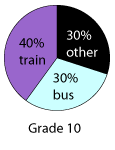Example 9: If Classes A and B each contain the same number of students and 30% of students in Class A are boys and 40% of students in Class B are boys, what percent of Classes A and B combined are boys?

 Thinking Here there are 2 equal groups of students, Class A and Class B. I know that 30% of students in Class A are boys and 40% of students in Class B. If both classes had 100 students, there would be 30 + 40 boys altogether out of the total of 200 (= 100 + 100) students. 70 out of 200 is the same as 35 out of 100 (because 70/200 = 35/100 are equivalent fractions). So we can say that while 30% of students in Class A are boys and 40% of students in Class B are boys, 35% of Classes A and B combined are boys.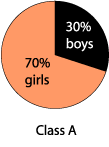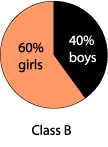In the first example, example 8, we are referring to one group of students so we can add the percents of students using the bus and train to get to school to get the percent of students using public transport.

In the second example, example 9, we are talking about two equal groups of students.

NOTE: A common mistake is to add percents for groups which aren't equal. This is not correct. When we want to find the combined percent of more than one group, where the groups are of different size, we need to take into account the proportions of the groups.

Example 10: If 30% of students in Class A are boys and 40% of students in Class B are boys, and Class A has 30 students in total and Class B has 20 students, what percent of Classes A and B combined are boys?

 Thinking Here Class A and Class B do not have the same number of students. I have to convert the percentages to numbers, add the numbers and then convert back to a percent. In this case Class A has 9 boys and Class B has 8 boys. Altogether, there are 17 boys out of a total of 50 pupils. This is 34% of the combined classes.Further Applications

Making comparisons

Although most people understand the concept of percent, they still get confused when working with more complicated percent problems, particularly those which ask us to compare the percents of different quantities. The following examples work through these types of problems.

Example 11: Comparing percents.
I have a 2 litre carton of milk of Brand A which is 95% fat free and 1 litre carton of Brand B which is 98% fat free, if I pour one glass of each, which glass of milk contains more fat?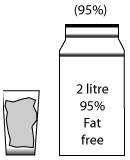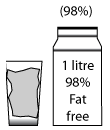Brand A Brand B

 Thinking Although the 2 litre carton of milk contains more milk than the 1 litre carton the fat free percent is 95%. This means that in the 2 litre carton there are 95 parts out of 100 parts that do not contain fat (or 5 parts out of every 100 which do contain fat). The 1 litre carton is 98% fat free. This means that in this carton 98 parts out of 100 parts do not contain fat (or 2 parts out of every 100 do contain fat). If we are comparing 2 glasses of milk, the glass containing Brand A milk will have 95 parts out of 100 fat free and the glass containing Brand B milk will have 98 parts out of 100 fat free. Therefore the glass of Brand A milk contains more fat.

Example 12: Finding the percent of combined quantities.

Lee has 2 different sized packets of coloured jelly beans: one is 100g and the other 250g. Each packet has 50g of black jelly beans. Lee makes up a bowl with both packets of jelly beans. What is the percent of black jelly beans in the bowl?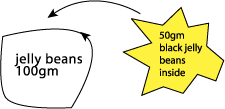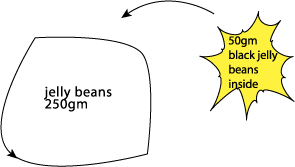Calculator short cuts

Simple electronic calculators with a percent sign can be very handy for adding percents. These calculators usually offer a shortcut method.

Example 13: Using a calculator to find 110% of 57

 Working Out Thinking Using my calculator, Enter 57 Press x Enter 110 Press % Answer: 62.7 To find 110% of 57 I can key in 57 x 110 % Note that the % key tells the calculator to work out an answer: it is analogous to the = key.

The more elementary the calculator the more likely a shortcut method is offered, but it will vary from calculator to calculator. Scientific calculators may not have this shortcut. You will notice that they usually do not have a '%' sign and you have to work out the problem by constructing a proper mathematical sentence.

NOTE: The method will depend on the calculator - you will need to experiment!

Example 14: Using a calculator to add 5% of 30 to 7% of 45

 Working Out Thinking Using my calculator, Enter 30 Press x Enter 5 Press % to calculate Press M+ to add to memory Press CE to clear screen Enter 45 Press x Enter 7 Press % to calculate Press M+ to add to memory Press MR to read answer Answer: 4.65 5% of 30 I can key in as 30 x 5 %. I can then use the memory function to store this answer 7% of 45 I can key in as 45 x 7 %. I can then add this to the memory and recall the final memory to see the answer. (NOTE: If you are not sure how to use the memory function of a calculator, see the section Whole Numbers, Multiplication, Using a calculator.)

Example 15: Using a calculator to increase \$120 by 7.2%

 Working Out Thinking Enter 120 Press + Enter 7.2 Press % Answer: \$128.64 This is a special short cut for adding a percent of a number. Check that it works on your calculator.

Example 16: Using a calculator to take a 15% discount from \$850.

 Working Out Thinking Using my calculator, Enter 850 Press - Enter 15 Press % Answer: \$722.50 Again this is a special shortcut. Check that it works on your calculator before you rely on it.Quick quiz

 1. A long distance bus which travels from Melbourne to Sydney has 45 passenger seats. (a) If 80% of the seats have been booked, how many seats is this? (b) If only 19 seats have been booked, what percent of seats is this? 2. There are 13 girls in Sari's class which make up 45% of the whole class. How many students are in Sari's class? 3. In an exam, Georgie answered 35 out of 40 questions correctly. (a) What percent of the questions did she answer correctly? (b) If the pass mark is 85%, did Georgie pass? (c) If Georgie resat the test and now scored 97%, how many questions did she answer correctly? 4. If a puppy put on 300 grams over the last week and this is 15% of its total body weight, what does the puppy weigh now? 5. Ryan bought 15 concert tickets to sell to his friends. (a) So far he has sold 5 tickets to his friends, what percent has he sold? (b) Ryan marked up the price of each ticket by 10% to pay for his own ticket. If he paid \$65 for the tickets originally, how much is he selling them for now? (c) Ryan has not had much success at selling the tickets and now has to discount them to get rid of them. If he discounts the price of \$65 by 20%, how much do the tickets cost now? (d) Eventually Ryan managed to sell 73% of the 15 tickets, how many did he sell? 6. Vinh purchased a pair of jeans and a T-shirt that were on sale at a 25% discount. The original price of the jeans was \$79 and the T-shirt was \$35. How much did Vinh pay for each item? 7. In a city office of 35 workers, 14 catch public transport to work, 8 ride their bikes, 10 drive their cars and 3 walk. (a) What percent of workers ride their bikes to work? (b) What percent catch public transport? (c) What percent drive or walk? (d) What percent use any method other than car to get to work? (e) If these figures were used as the basis of a transport survey how many people out of 100 could be said to use each method of transport to get to work? 8. One brand of pumpkin soup weighs 440 gs and contains 35% fat. Another brand weighs 1 kg and contains 20% fat. If I have a bowl of each, which bowl of soup contains more fat?